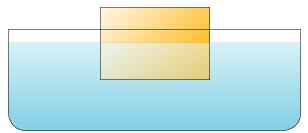# Problem: A rectangular wooden block of weight W floats with exactly one-half of its volume below the waterline.A. What is the buoyant force acting on the block?B. The density of water is 1.00g/cm3 . What is the density of the block?

###### FREE Expert Solution

A. The net force on the block when it is just floating is zero.

Therefore, the buoyant force is equal to the weight of the block.

90% (441 ratings)###### Problem Details

A rectangular wooden block of weight W floats with exactly one-half of its volume below the waterline.A. What is the buoyant force acting on the block?

B. The density of water is 1.00g/cm3 . What is the density of the block?